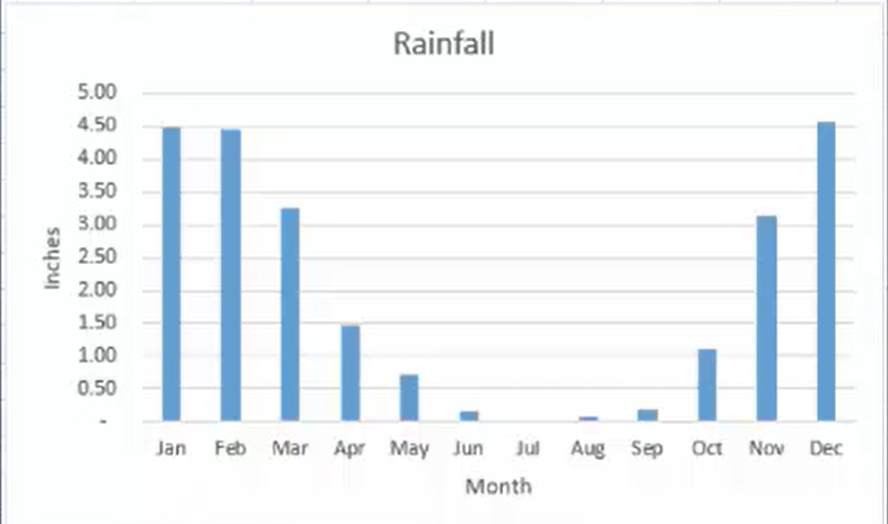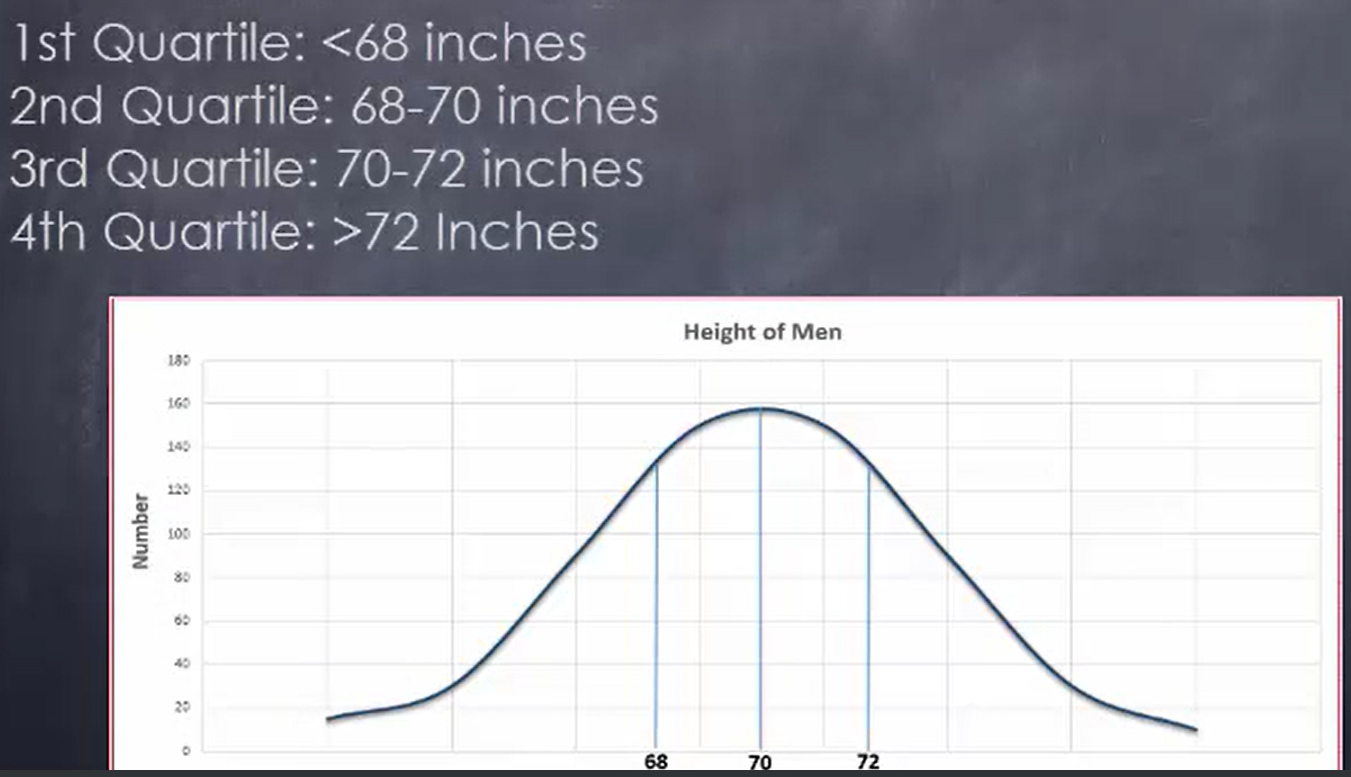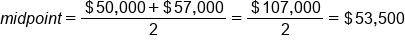+
Calculating the Interquartile Range
1 Tutorials that teach Calculating the Interquartile Range
Take your pick:

# Calculating the Interquartile Range

##### Rating:
(5)
• (1)
• (1)
• (1)
• (0)
• (2)
Author: Sophia Tutorial
##### Description:

Calculate the interquartile range of a given set of data.

(more)
Tutorial
what's covered
This lesson discusses calculating the range of data. You will be able to determine both the range and the interquartile range of a data set. This lesson covers:
1. Variability in Data
2. Range
3. Interquartile Range

## 1. Variability in Data

In addition to finding the center of a data set, such as the mode or median, statistics is also interested in finding a number that tells you how far the data set is spread out from the center. By determining how spread out the data set is, you are establishing a measure of what is known as variability. Two ways to measure variability are the range of a data set and the interquartile range of a data set.

With either of these measures, understanding the variability in data can help determine if the findings of two tests are comparable. Tests may come from within the same study or may be from studies conducted at different places or at different times. By comparing variability of different tests or studies, you can add reliability to those tests or studies. When there is less variability between data across the test or studies, these tests may be considered more valid.

If you recall from the eight steps of the experimental method, step seven asks to revise the guess if the prediction is wrong, and return to step two. If the prediction looks right, you need to start testing again from step four to verify the results.

think about it
Why would you want to keep testing?

As you continue testing, you help assure a minimal degree of variability in the test, which in turn helps validate the results of the test that you’re conducting.

## 2. Range

Finding the range of a data set means finding the smallest value, known as the minimum, and the largest value, which is known as the maximum. The range is simply the difference between the maximum and the minimum values. The range is a measure of variability or spread, meaning that the larger the range, the more spread out the values in the data set are.

hint
Keep in mind that if the data set has extreme values, the range may not accurately represent the spread of the data.

Say you’re dealing with incomes of people, a situation where there’s going to be a very large range. You could have very low income, maybe a few thousand dollars a year, and you could have a very high income, potentially tens of millions of dollars per year. Obviously, this is an extreme range. It’s not going to give you a good sense of how well those incomes are distributed.

Take a look at this graph of the average monthly rainfall in San Francisco:think about it
How do you figure out the range of this particular data set?

Arrange the numbers from smallest to largest. July has the least amount of average rainfall with zero inches. In December, they received 4.57 inches. Take the difference, which is 4.57, and that is your range.
term to know
Range
The difference between the maximum and minimum values
formula
Range
Maximum Value - Minimum Value

## 3. The Interquartile Range

Sometimes, however, you might be interested in determining how an observation falls into a specific range in order to make comparisons to other observations. In some cases, splitting your information into groups called quartiles, which each represent 25% of the data, can be useful.

A chart of men’s heights will have a normal distribution. The median height for men, as shown below, is roughly 70 inches. The quartiles are broken down here:According to this, anything less than 68 inches will be in the first quartile, anything between 68 and 70 inches will be in the second quartile, between 70 and 72 in the third, and anything above 72 inches will be in the fourth quartile.

When considering just the range of a data set, sometimes the range can be thrown off by an occasional random value that may cause the range to not represent the true variability of a data set. In a case such as this, it’s useful to have a measure of variability that is more accurate. Such a measure of variability is known as the interquartile range.

While the range represents the entire extent of the data set, the interquartile range represents the middle 50% of the data set. Like the range, the interquartile range is a measure of variability or spread. The larger the interquartile range, the more spread out the values in the middle 50% of the data set are. The interquartile range is a more reliable measure of the spread of data than the range is, because it does not only take into account the maximum and minimum values of a data set.

To find the interquartile range of a data set, find the first quartile (Q1) and the third quartile (Q3). The interquartile range is then the difference between the third quartile and the first quartile. To consider the middle portion of the data, you are only concerned about the first and the third quartile.

formula
Interquartile Range (IQR)
Q3 - Q1

So how do we go about figuring this out?

First, sort the data from smallest to largest value, and then, divide it into two halves. The middle value would be the median. Next, find the middle value of the first half. This is the first quartile, Q1. Third, determine the middle value of the second half. This would be the third quartile, or Q3. Finally, subtract Q1 from Q3.

Let’s look at a hypothetical data set that involves the income of 25 to 34 year olds:

Income of 25 to 34 year olds
\$20,000 \$57,000
\$27,000 \$61,000
\$29,000 \$62,000
\$32,000 \$66,000
\$33,000 \$71,000
\$36,000 \$77,000
\$40,000 \$84,000
\$43,000 \$88,000
\$45,000 \$96,000
\$48,000 \$102,000
\$50,000 \$107,000

1. Sort the data from smallest to largest value and divide it into two halves.

The data is already listed from the smallest value of \$20,000 to the largest value of \$107,000. This data set has an even number of values (22 total). When dividing this data into two halves, the data will actually split right between \$50,000 and \$57,000. So the midpoint will actually be between these two points, or \$53,500.

Income of 25 to 34 year olds
\$20,000 \$57,000
\$27,000 \$61,000
\$29,000 \$62,000
\$32,000 \$66,000
\$33,000 \$71,000
\$36,000 \$77,000
\$40,000 \$84,000
\$43,000 \$88,000
\$45,000 \$96,000
\$48,000 \$102,000
\$50,000 \$107,000

The midpoint of an evenly numbered data set can easily be found by adding the two middle values and dividing by 2:2. Find the middle value of the first half. This will the first quartile or Q1.

The first half of the data are the 11 values from \$20,000 to \$50,000. The middle of the first half is at \$36,000, also known as Q1.

Income of 25 to 34 year olds
\$20,000 \$57,000
\$27,000 \$61,000
\$29,000 \$62,000
\$32,000 \$66,000
\$33,000 \$71,000
\$36,000 (Q1) \$77,000
\$40,000 \$84,000
\$43,000 \$88,000
\$45,000 \$96,000
\$48,000 \$102,000
\$50,000 \$107,000

3. Find the middle value of the second half. This will the third quartile or Q3.

The second half of the data are the 11 values from \$57,000 to \$107,000. The middle of the second half is at \$77,000, also known as Q3.

Income of 25 to 34 year olds
\$20,000 \$57,000
\$27,000 \$61,000
\$29,000 \$62,000
\$32,000 \$66,000
\$33,000 \$71,000
\$36,000 \$77,000 (Q3)
\$40,000 \$84,000
\$43,000 \$88,000
\$45,000 \$96,000
\$48,000 \$102,000
\$50,000 \$107,000

4. Subtract Q1 from Q3, or Q3 - Q1.

Using this data set, we know the median, or Q2, is equal to \$53,500, Q1 is equal to \$36,000, and Q3 is equal to \$77,000. So the interquartile range for this group of income is equivalent to \$77,000 - \$36,000, or \$41,000.

Notice how that compares to the range, which is \$87,000. That is twice as much as the interquartile range. You can see how the interquartile range gives us a much better measure of variability.

term to know
Interquartile Range (IQR)
Represents the middle 50% of the data set

summary
You looked at what variability in data can mean and its importance. You also learned how to determine the range of a data set. After that, you learned how to calculate the interquartile range of a data set, which is a better measure of variability.

Source: This work is adapted from Sophia author Dan Laub.

Terms to Know
Interquartile Range (IQR)

Represents the middle 50% of the data set

Range

The difference between the maximum and minimum values

Formulas to Know
Interquartile Range (IQR)

Q3 - Q1

Range

Maximum Value - Minimum Value

Rating Header